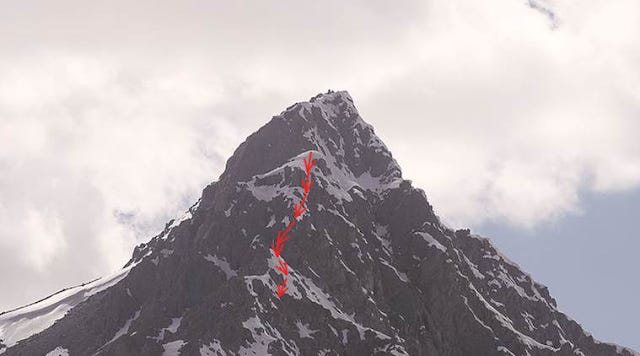# An introduction to Gradient Descent

Gradient Descent is the most popular optimization strategy, used machine learning and deep learning right now. It can be combined with almost every algorithm yet it is easy to understand. So, everyone planning to go on the journey of machine learning should understand this.

### Introduction

Gradient descent is used to find local minima of a given function. So, It is a convex function based optimization algorithm.

It is simply used to find the values of the parameters at which the given function reaches its nearest minimum cost.

We start by defining initial parameters and then with derivation of the function, we adjusts the values of parameters iteratively.

### Intuition

“A gradient is the ratio which relates the input and output of a function. How small changes drives changes in the output of the function.”

Suppose we have a function f(x) = x^2. Then the derivative of the function is 2*x.

It means if the x is changed 1 unit then f(x) will change 2*1

Think of a person trying to descent a hill, blindfolded. He want to reach down with minimum cost(steps). What he can do is that he take steps in the steepest direction and from there again search the steepest curve and move in that direction. As he reach near to flat surface the steepness of the surface becomes flat(the local minima). So, his steps becomes smaller.

Illustration of steps on Tiger hill.

### Math proving this

The equation below shows how it’s done: ‘x(next)’ is the new position of the person, ‘x(current)’ is the current position, subtraction means that we ‘gamma is the step and ‘nabla f(x)’ is the gradient showing the steepest direction.

This shows us we need to move in which direction, how much to minimize the function.

Let’s take another example of Linear regression technique in machine learning, We have to find optimal ‘w’ and ‘b’ for the cost function J(w, b) where J is minimum.

below is an illustration of convex function, (w and b) are represented on the horizontal axes, while J(w, b) is represented on the vertical axis.

We start by taking random values for w and b somewhere near the top and then the gradient descent then takes small steps towards steepest direction down till it reaches the point where the cost function is as small as possible.

### How big steps to take(Learning rate)

The steps which are taken to reach optimal point decides the rate of gradient descent. It is often referred to as ‘Learning rate’. To reach the local minima efficiently, we have to set them appropriately, neither too high nor too low.

This is because if the steps(learning rate) are too big, it bounce between the convex function and may not reach the local minimum. If the learning rate is too small then gradient descent will eventually reach the local minimum but it will take too much time for that.

To make sure Gradient Descent is working properly, we can plot(number of iterations vs. Cost function) the cost function as Gradient Descent iterates. This allows us to easily check how good our learning rate is. Just try and put all together.

The cost function should decrease overtime if the Gradient Descent is working properly.

When Gradient Descent can’t reduce the cost function anymore and remains near the same level, we can say it has converged. The number of iterations for convergence may vary a lot.

If your learning curve is just going up and down without reaching a lower point, you should try decreasing the learning rate. Just simply try with 0.001, 0.003, 0.01, 0.03, 0.1, 0.3 etc. and see which is better.

### Types of Gradient Descents

#### 1. Batch Gradient Descent

Also called vanilla gradient descent, calculates the error for each example in the training dataset, the model is updated only after all examples have been evaluated. This is called one epoch where all training examples have been evaluated.

#### 2. Stochastic Gradient Descent

Stochastic Gradient Descent (SGD) unlike vanilla, iterates over each training example in the dataset while updating the model. The thing is that frequent updates can be computationally more expensive.

#### 3. Mini Batch Gradient Descent

This is the method having a combination of concepts of both SGD and Batch Gradient Descent. It splits the dataset into small batches and then performs update on each of these batches balancing between the efficiency of batch gradient descent and the robustness of SGD.

Source: Deep Learning on Medium